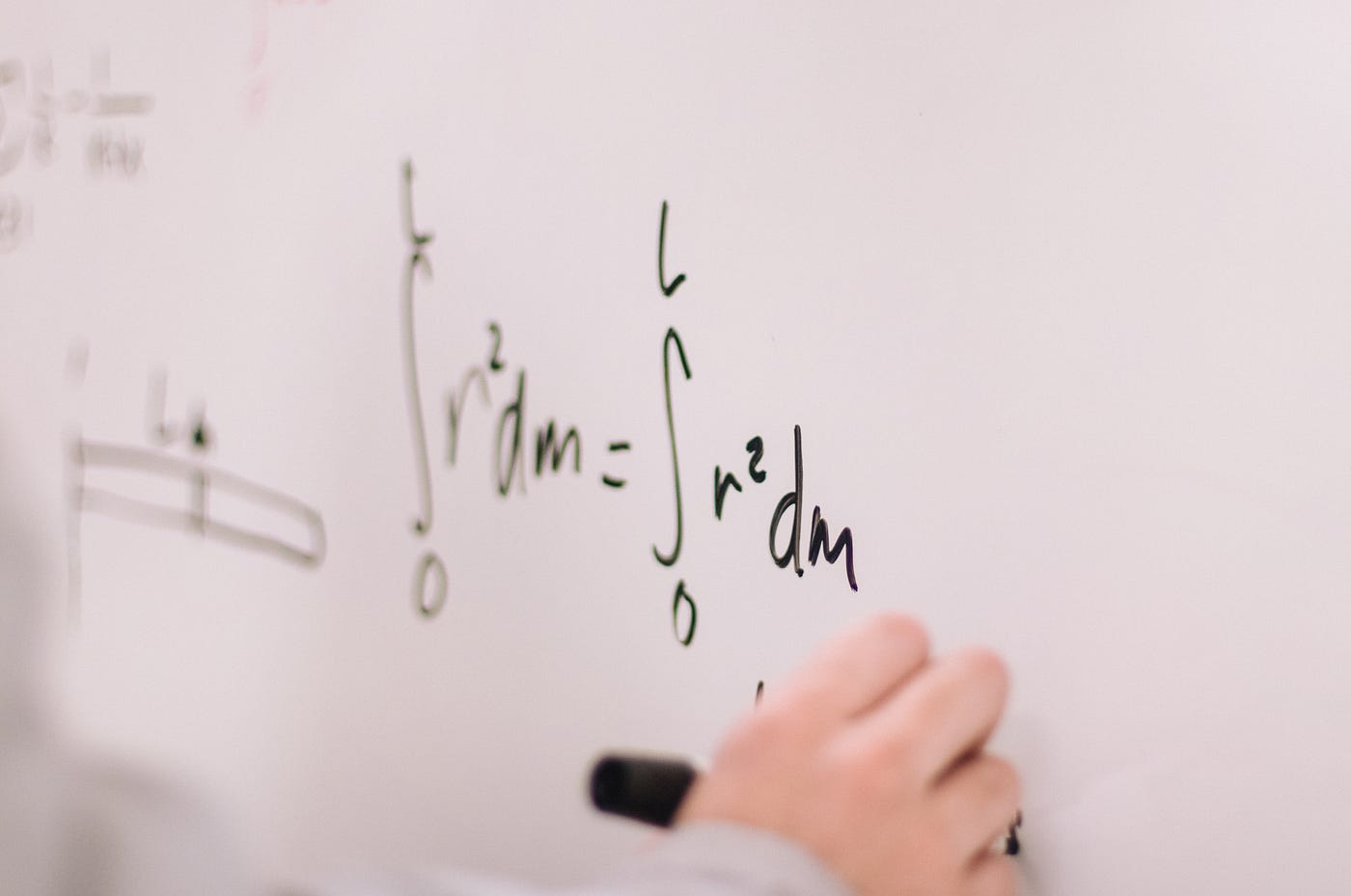# Solutions to Ordinary Differential Equations of First OrderPhoto by Jeswin Thomas on Unsplash

There are so many aspects a person has to keep in mind while solving differential equations that you’re bound to forget something at some point. This can be very frustrating as by making a small mistake, differential equations can become unsolvable. The purpose for writing this is to create a helping guide to help remember everything.

# Variable Separable ODEs

I’ll start with variable separable differential equations. Although a variable separable ODE should be in the form

dy/dx = g(x)h(y)

which you can easily solve by applying algebraic operations and converting it into the form

h(y)dy = g(x)dx

and then finally integrating both sides to obtain the solution. This is rarely the case and the ODE we generally deal with are not provided to us in such a simple form. To solve these equations, just apply algebraic operations to separate the variables and bring it into the form mentioned above and integrating it.

# Linear Differential Equations

There are two types of linear equations, homogenous and non-homogenous differential equations. These equations are generally in the form

a(x) dy/dx + b(x)y = g(x).

The reason why these equations are called linear is because the dependent variable(y) has a power of one. To solve them, we first have to bring them in the form

dy/dx + P(X)y = f(x)

If f(x) in this form comes out to be zero, it’s called a homogenous differential equation, otherwise it is a non-homogenous differential equation.

To solve these, we first find the integrating factor. We do this by integrating P(x) and setting it as the power of e. We then multiply the integrating factor[IF] with the entire equation giving us the equation in the form

[IF]dy/dx + [IF]P(x)y = [IF]f(x)

which is equivalent to

d/dx [IF] . y = [IF]f(x)

Integrating both sides should give us the solution.

# Exact Equations

The criteria for an equation to be exact is that if we write it in the form

M(x,y)dx + N(x,y)dy = 0

the partial derivative of M with respect to y and the partial derivative of N with respect to x should be continuous that is

∂M/∂y = ∂N/∂x

Once it is established that the equation is exact, for the solution we apply integration in the form

∫ M dx + ∫ (Terms of N free from x) dy = C

or

∫ N dy + ∫ (Terms of M free from y) dx = C

after integration we can obtain the solution for the equation.

Even if a differential equation is non-exact, we can make it exact by finding its integrating factor and multiplying with it. We find its integrating factor by taking the partial derivatives and putting them in the formula

P(x) = (∂M/∂y - ∂N/∂x)/ N

or

P(x) = (∂N/∂x - ∂M/∂y)/ M

we then integrate P(x) and set it as the power of e. We then multiply both M and N by the integrating factor to make the differential equation exact and then test it for exactness again by finding the partial derivatives.

# Homogenous Differential Equations

To solve a homogenous differential equation, we first have to perform a homogeneity test. We do this by solving the equation by putting the values of x and y as λx and λy respectively. If the equation comes out in the form where

M(λx, λy) = λªM(x, y)

and

N(λx, λy) = λⁿN(x, y)

where n is the degree of homogeneity. Once it is verified that the given equation is a homogenous differential equation, we come towards solving it. To solve it we perform a substitution of either y = ux or x = uy. We then take the derivative of this substitution and write it in the form

dy = u dx + x du

if we take the example of y = ux. We then perform the substitutions to bring the equation in the form where the variables are u and x. We then perform the integration to obtain our solution in the form of u and x. We resubstitute the value of u to obtain our final solution.

# Bernoulli’s Equations

The differential equations in the form

dy/dx + P(x)y = Q(x)yⁿ

where n is zero, is called a Bernoulli differential equation. If n = 1, it can also be said to be a Bernoulli differential equation as it can be written in the form

dy/dx + [P(x)y-Q(x)yⁿ] = 0

To solve Bernoulli equations, we first divide the entire equation by yⁿ to obtain

y ⁻ⁿ dy/dx P(x) y ⁻ⁿ = Q(x)

and then make the substitution where

u = y¹⁻ⁿ

By using chain rule we get

du/dx = (1 - n)y⁻ⁿ. dy/dx

which can also be written as

y ⁻ⁿ. dy/dx = du/dx . 1/(1 - n)

After substitution we get

du/dx . 1/(1 - n) + P(x)u = Q(x)

By algebraic manipulation we get

du/dx + (1 - n)P(x)u = (1 - n)Q(x)

which can be solved as a linear differential equation linear in u. Once a solution is obtained in u, u can be re-substituted to obtain a solution in y.

--

--